### IMO Shortlist 2011 problem A6

Kvaliteta:
Avg: 0,0
Težina:
Avg: 8,0
Let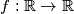$f : \mathbb R \to \mathbb R$ be a real-valued function defined on the set of real numbers that satisfies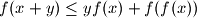for all real numbers$x$ and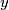$y$. Prove that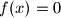$f(x) = 0$ for all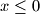$x \leq 0$.

Proposed by Igor Voronovich, Belarus
Izvor: Međunarodna matematička olimpijada, shortlist 2011